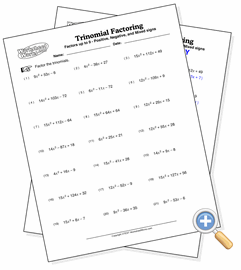# Trinomial Factoring

## Composite Variable CoefficientsDevelop trinomial factoring skills involving one or more coefficients

These are trinomial factoring problems where one or both of the second degree term has a coefficient. The coefficients make the problem significantly more difficult by increasing the number of potential combinations needed to find the factors. However, there is still only one solution to each problem.